## Monday, December 27, 2010

### The big book of brain games: a puzzle

Ultimate spoiler:A record solution that TRF reader bbzippo found a few years ago: 23 extra linkages, no intersections. Only previous record holders are discussed in detail here: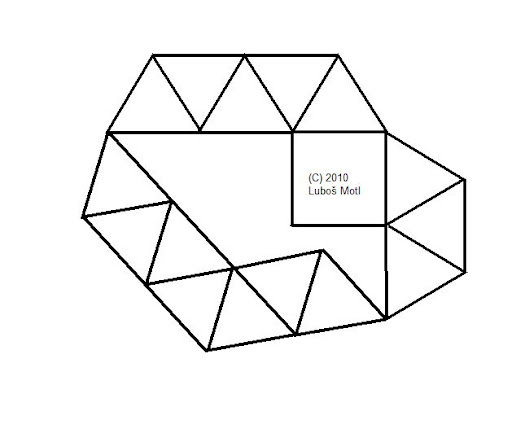This solution of mine uses 31 extra edges or, if you allowed frictionless intersections and if you could shorten the lower left detour by one small triangle, it would use 29 extra edges. Go to the end of the article for comments why this is a valid solution.

Phil Gibbs' blog that I regularly read and learn from - and that actually doesn't irritate me in any way - posted a very cool Christmas puzzle from “The Big Book of Brain Games” by Ivan Moscovich. Most of the puzzles are claimed to be easy but puzzle number 331 is subtle and very interesting:
Draw a square consisting of four equally long connecting line segments hinged at the vertices. Such a structure may degenerate into a rhombus if you apply some pressure. How many additional interlinks of the same length must be supplemented to prohibit this excessive degree of freedom and to prevent the square from being tilted? The interlinks must belong to the same plane as the quad and each one may only be pegged to others at the endpoints.
So far, I can't link to the original blog now because you would find a solution. The owner of the blog and his fastest reader found a solution with 43 extra linkages. After I was told what it was, your humble correspondent found a generalized solution of the same kind that only uses 29 extra linkages, if you allow me to draw frictionless intersections, or 31 extra linkages, if you don't.

I recommend you to waste a few hours with drawing pictures whose faces have internal angles that are multiples of 30 degrees. It's useful because you will learn that one can waste a lot of time by drawing seemingly attractive structures if he or she makes a completely wrong assumption or too constraining an Ansatz about the solution. ;-)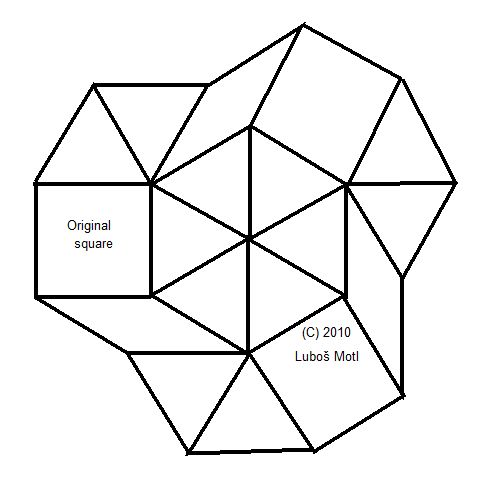An example of a solution attempt that doesn't work. Your humble correspondent drew many of them! :-)

It seems pretty clear to me that no solution of the kind that is describe in the previous big paragraph can exist. You must make the structure solid by thinking outside the box a little bit. :-) If it helps you, here is a Mathematica 7 code that allowed me to find my more nontrivial solution once I understood the broader concept that is actually promising:
finds = {{0, 0, 0, 0, 0, 0, 0}};

(* next, separated line with input *)
Dynamic[{a, b, lenVsq, c, d, e, f}]
Dynamic[MatrixForm[finds]]

(* next, separated line with input *)
For[a = 1, a <= 7, a++,
For[b = -7, b <= 7, b++,

v = {a, 0} + b*{1/2, Sqrt/2};
lenVsq = v[]*v[] + v[]*v[];

For[c = -5, c <= 5, c++,
For[d = -5, d <= 5, d++,
For[e = -5, e <= 5, e++,
For[f = -5, f <= 5, f++,

u = {d/2 + c, d*Sqrt/2}
- {f*Sqrt/2, f/2 + e};

lenUsq = u[]*u[] + u[]*u[];

finds =
If[(c*c + d*d)*(e*e + f*f) != 0 &&
Abs[lenUsq - lenVsq] < 0.00001,
finds~Join~{{a, b, lenVsq,
c, d, e, f}}, finds];

]
]
]
]
]
]

I guess that if you're not told the main idea, chances are that even the code above will be useless for you. Their "solution 43" as well as my "solution 29/31" have already been posted to the web but I won't give you coordinates before some of you try to solve it - and perhaps find an even more economical solution?

Bonus: a no-go theorem

I am sure that I was not the only one who got stuck for some time with tests of pictures like this one:Well, JollyJoker is another victim because the picture above was offered by him.

Note that there is a whole class of pictures where all the linkages are either vertical, or horizontal, or have another azimuthal angle that is a multiple of 30 degrees. Correspondingly, all internal angles of all faces are multiples of 30 degrees as well - 30, 60, 90, 120, or 150 degrees.

With some help from TRF, it's straightforward to prove that no picture of this kind can be rigid.

First, use (0,0), (1,0), (0,1), (1,1) for the vertices of the square. If all edges have one of the allowed azimuthal angles mentioned above - 0, 30, 60, 90, 120, or 150 degrees (or the opposite-direction edges whose azimuthal angle differs by 180 degrees), then all vertices or hinges indirectly connected to the square have coordinates that are integer linear combinations of the following four vectors:
t = (1, 0)
u = (1/2, sqrt(3)/2)
v = (0, 1)
w = (sqrt(3)/2, -1/2)
Note that the nontrivial numbers are, up to the last sign, sines and cosines of 30 or 60 degrees. However, if you have a collection of points whose coordinates are
at + bu + cv + dw,
a, b, c, d are integers,
then you can see that both coordinates of the points are integer combinations of 1/2 and sqrt(3)/2. However, you may deform the picture by preserving vectors t,u and rotating v,w into v',w':
v' = (sin(phi), cos(phi))
w' = (sin(120°+phi), cos(120°+phi))

point' = at + bu + cv' + dw',
a, b, c, d are integers.
All edges whose length was equal to 1 in the old diagram - composed of points with coordinates point - have to have the length 1 in the new diagram composed of points with coordinates point'. That's because
t, u, t-u; -t, -u, -t+u;
v, w, v+w; -v, -w, -v-w
are the only integer combinations of vectors t,u,v,w whose length is (or was) exactly equal to one (see below). However, if you replace v,w by v',w' in the expressions above, it's still true that all the vectors will have length equal to one. That proves that the diagram may be tilted.

To show my lemma, recall that the general linear combinations of the vectors t,u,v,w may be written as
{ (A+B sqrt(3))/2, (C+D sqrt(3))/2 }
Four times the squared length of this vector should be equal to 4 but it is
+ A2 + 3B2 +
+ C2 + 3D2 +
2 sqrt(3) (AB+CD)
where A,B,C,D are integers. This can only be equal to 4 if (AB+CD) vanishes - it's the coefficient of the square root of three, an irrational number that can't cancel against others because of the integrality of the coefficients. Moreover, the four positive terms on the first two lines have to add up to four.

It may only happen if this number four arises as 4+0+0+0 or 0+0+4+0 or 1+0+0+3 or 0+3+1+0 - all higher numbers are easily seen to exceed 4. Moreover, 1+3+0+0 and 0+0+1+3 are also forbidden because AB+CD would be nonzero (plus minus three). That proves that there are only 6 possible vectors and their 6 opposite vectors whose length is equal to one. In terms of the old basis, they were written as
t, u, t-u; -t, -u, -t+u;
v, w, v+w; -v, -w, -v-w
You see that the combinations of t,u are decoupled from the combinations of v,w, so you may rotate v,w separately from t,u (that you may keep fixed, for example), and it won't break any of the linkages because all linkages that existed will continue to have length equal to one.

This no-go theorem is an example of the fact that you may get stuck in drawing seemingly pretty pictures and you may hope that if you combine the flowers in a cleverer way than an hour ago, you will succeed. However, it can be shown that the whole infinite class of such pictures is ruled out.

The same thing holds in many other contexts. In particular, all people working on discrete theories of spacetime - or any quantum theory of gravity that is not equivalent to string theory - may spend lots of time (it's decades or centuries rather than hours in this case) by fabricating more convoluted models.

But it can be seen that there are no consistent non-stringy theories of quantum gravity. The latter statement and its proof are more complex than the example above - and the proof arguably demands a lot of knowledge from the readers - but the statement is equally true as the no-go theorem for solutions to the linkage problem whose angles are multiples of 30°.

You need to add a new player - internal angles that are not multiples of 30 degrees, in this case - to have a chance to find a solution. Then you enter a broader, different set of candidates - the counterpart of the string theory landscape - and in this set of candidates, which encourages you to study different issues than in the wrong class and to generalize them in different ways than before, you may actually construct correct and/or minimal solutions to your problem.

Spoilers: best solutions with 31 extra edges

The best solution available at this moment was found by your humble correspondenent by refining the Pythagorean idea. The Pythagorean solution was independently found by Phil Gibbs, Bill K, and Honza U. who was the first successful TRF reader to solve the challenge: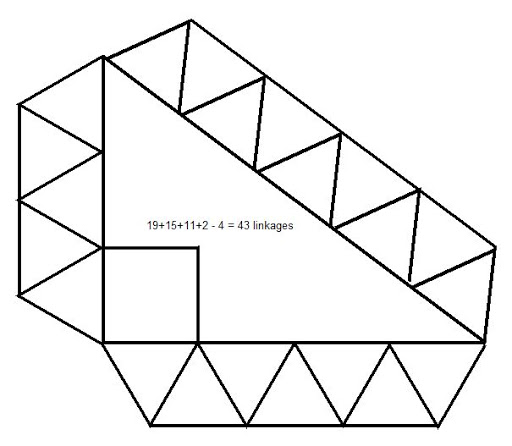The solution uses the Pythagorean identity 3^2+4^2=5^2. The three "rigid bridge elements" contain 19, 15, 11 linkages, respectively, and 19+15+11+2-4 = 43 new linkages. The number doesn't change if you allow self-intersections (a point that was misinterpreted by Honza).

Mr/Ms Imho cannot be counted as a successful solver because he or she thought that you can erase the whole bridge elements and preserve just the 3+4+5 thin linkages surrounding the large 3-4-5 Pythagorean triangle (which would mean 12-2=10 extra linkages). Well, indeed, such a construction wouldn't be rigid at all and I hope that Mr/Ms Imho is not a professional architect. :-)

However, using the Mathematica code above, I found a more efficient solution that only uses 31 extra line segments:or, if you allow frictionless intersections of the line segments, 29 extra linkages: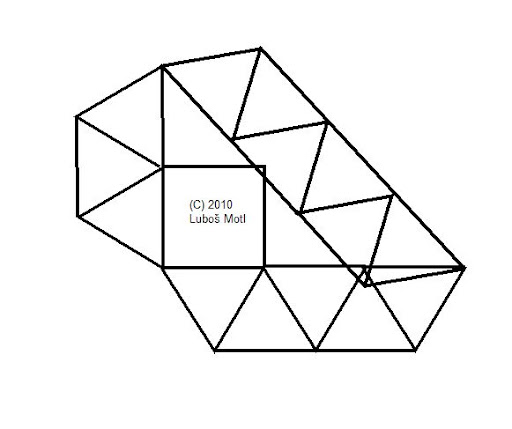It is similar to the 3-4-5 Pythagorean triangle except that the catheti are not 3 and 4 but rather 2 and 3. You may complain that the hypotenuse is not an integer. Indeed, it's not: its length is sqrt(2^2+3^2) = sqrt(13).

However, one can create a rigid line segment of length sqrt(13) using the equilateral triangular truss, too. Draw a triangular truss that includes horizontal lines and the point (0,0). Make three steps to the East (right): the coordinate of the final point will be (3,0).

Now, make a step to the (almost) Northeast. You will add (1/2, sqrt(3)/2) to the coordinates of your point so the final point will be
(3.5, sqrt(3)/2)
The squared length of this vector - the distance between the two points of the triangular truss - equals
3.52 + 3/4 = 12.25 + 0.75 = 13
just like previously. So three pieces of the triangular bridge construction may be connected just like in the case of the 3-4-5 Pythagorean triangle and the right angle of the square is ensured in this way.

The Mathematica program above, which has only searched through a set of sufficiently "small" solutions, has also found a solution with the hypotenuse equal to sqrt(39). In that solution, one has to use both directions of the triangular trusses on all three sides of the big triangle.

Exhaustive search: a proof of minimality

It's actually not hard to find the 29/31 solution above in a controlled, exhaustive search for economical solutions. Earlier in this text, I showed that there must exist internal angles that are not multiples of 30 degrees. Obviously, there must exist at least two such hinges where the internal angles not divisible by 30 degrees exist.

At least two such hinges are indirectly connected to the square by some linkages. Look at these two hinges. Of course, in the minimum setup, there will be exactly two such linkages.

The assumption in the previous sentence was proved to be wrong. The current most economical solution known to me - at the top of the article - depends on four hinges whose internal angles differ from multiples of 30 degrees. This fact invalidates the rest of the proof but it may still be interesting for you to read it.

To fix the angles of the square, the distance between these two linkages must be protected by an additional network of linkages - which will become the "hypertenuse" in the Pythagorean-based solutions. It's almost guaranteed that the minimal structure that preserves the distance is made out of a triangular truss.

Now, you may enumerate all the squared distances you may obtain from a triangular network. If you classify them by the number of "Northeast" steps, the allowed squared distances are:
0 NE steps: 0, 1, 4, 9, 16, 25, ...
1 NE step: 1, 3, 7, 13, 21, 31, ...
2 NE steps: 3, 4, 7, 12, 19, 28, ...
3 NE steps: 7, 9, 13, 19, 27, ...
4 NE steps: 12, 13, 16, 21, 28, ...

union: 0, 1, 3, 4, 7, 9, 12, 13, 16, ...
The last line will be referred to as the white list.

Note that all of the squared lengths are integers. These are the allowed squared lengths of the "hypertenuse" block that preserves the distance between the two hinges. Now, the two hinges are connected to the square, so their coordinates have to differ by an integer combination of the vectors t,u,v,w mentioned previously.

Let's redo an exercise we did in a different basis. A general integral combination of the vectors t,u,v,w - the vector difference between the two hinges, as calculated from the block containing the square
At + Bu + Cv + Dw
has the squared length equal to
A2 + B2 + C2 + D2 +
+ AB - CD + sqrt(3) (AD + BC)
There are no AC and BD terms because the pairs t,v and u,w are orthogonal: even in the upper part of the text, I have switched the sign of "1/2" in w to "-1/2", apologies to old readers. ;-)

Now, because the squared distances that can be supported by the "hypertenuse" blocks are integers, it follows that AD+BC has to vanish. Consequently, it can't be true that exactly three of the numbers A,B,C,D are nonzero: either 4 or 2 (or fewer) are nonzero. If 2 (or fewer) numbers among A,B,C,D are nonzero, we have either B=D=0 or A=C=0, which returns us to the Pythagorean numerology and 3-4-5 is the smallest solution (optimization of the 3-4-5 triangle may be discussed separately) , or A=B=0 or C=D=0 which are not allowed because the construction would only be attached to one of the sides of the square.

If all A,B,C,D are nonzero, which is the only room for solutions that may differ from the Pythagorean 3-4-5 concept, we have to check the combinations
A,B,C,D = K,L,K,-L
A,B,C,D = K,K,L,-L
K,L = 1,1 or 1,2 or 2,2 or 2,3
and their equivalents with sign flips and permutations that don't change the essential geometry (and the new angles at the special hinges). The apperance of K,L = 3,3 or more or K,L = 2,4 or more would already produce the total squared length above 31. We must also check
A,B,C,D = 1,2,2,-4.
However, it produces the squared length of 1+4+4+16+2+8 = 35 or 1+4+4+16-2-8 = 15 (for -1,2,2,4) which are not on the "white list" and are getting too high, anyway. Similar small values of A,B,C,D that are not pairwise equal may be seen to produce too big a squared length, or a squared length that is not in the allowed list. For example, for A,B,C,D=1,2,3,-6, one gets AD+BC=0. However, the squared length is 1+4+9+36+-(2+18)=50+-20 which is 30 or 70, too large.

That's why we have reduced the unknown yet promising solutions to the cases when all A,B,C,D are nonzero and expressed in terms of K,L as above. In the two inequivalent cases that make AD+BC vanish, namely K,L,K,-L and K,K,L,-L, the squared length of the vector (the sum of squares of A,B,C,D plus AB-CD) is equal to 2K^2+2L^2+-2KL or (3K^2+3K^2 or K^2+L^2).

The result must belong to the allowed list of squared lengths, 0, 1, 3, 4, 7, 9, 12, 13, 16, ... Clearly, the length 1 would only produce constructions with angles divisible by 30 degrees again which is no good, as proved at the beginning. Let's continue with the white list.

What about the squared length equal to 3, 7, 9, or 12?

The odd numbers can't be obtained as 2K^2+2L^2+-2KL because the latter is even. They can be written as K^2+L^2 or 3(K^2+L^2) but only if one of K,L vanishes... We want both K,L nonzero, as mentioned previously (because the construction would only be attached to one side of the square).

The smallest number of the form K^2+L^2 for positive integers K,L that is on the white list is 13 for K,L=2,3 or 3,2: numbers 2,5,8 are not on the white list. And 18 = 3^2+3^2 that would be just a little bit bigger is not on the white list, either.

The template 3(K^2+L^2) is clearly even less useful to create small solutions. The smallest allowed values of this tripled sum for positive integers K,L, namely 6,15,24..., are not on the white list, either. So far, K^2+L^2=9+4=13 is the only nontrivial small solution we found.

Finally, we must deal with the template 2(K^2+L^2+-KL); of course, the negative sign is better to produce smaller results. This can only match the even numbers on the white list, namely 4,12,16..., because it is even. K^2+L^2+-KL would have to be equal to 2,6,8... However, 2,6,8 can't be written in this form: 2,6 are equal to 2 modulo 4, but K^2+L^2+-KL can't be 2 mod 4. And 8 is also impossible for similar reasons.

So the only new small solution we found was one based on the hinges whose distance is sqrt(13).

Top intersecting solution

The assumption of just 2 vertices (hinges) that are not combinations of t,u,v,w is severely violated in the state-of-the-art best solutions. At the top, there is bbzippo's non-intersecting solution with 23 extra linkages.

Frictionless intersecting solutions can go down to incredible 15 extra linkages. This one is an example - a modified JollyJoker's structure: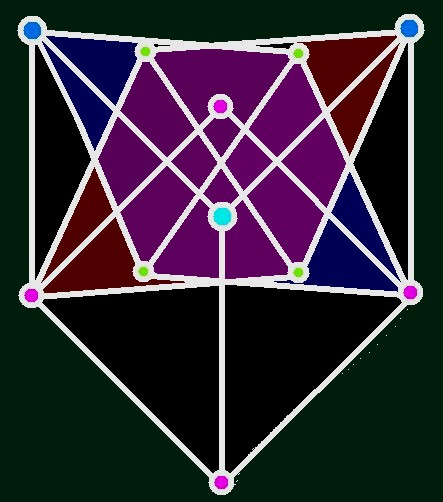Note that the original square with the pink vertices is rotated by 45 degrees. There are 3 additional - blue - vertices in the picture. Their 6 coordinates are constrained by 7 conditions on the length; this overdetermined system of conditions (by one) is just the right amount to fix the angle of the square with pink vertices.

The 7 distances that are fixed include the 3 vertical length-one distances from the adjacent pink vertices of the square; 2 length-one distances of the central light blue new point from the two dark blue new points on the sides; and 2 length-sqrt(3) distances of the upper blue points from the most distant pink vertices on the opposite side - that are realized by the di-triangle rhombuses.

This new solution brings you into an entirely new class of possibilities. Just add N vertices to the plane - outside the integer combinations of t,u,v,w - such that 2N+1 distances between these vertices (either between pairs of them, or between the vertices and arbitrary points in the "lattice" of integer combinations of t,u,v,w) belong to the white list (i.e. can be realized by inserting parts of triangular trusses).

Such a new template looks very general and it would be very time-consuming to look for all solutions, even pretty small ones, but I can still argue that even this general class actually doesn't exhaust all the possibilities. One could also "add the angles" etc.

#### 1 comment:

1.Mr/Ms Imho cannot be counted as a successful solver because he or she thought that you can erase the whole bridge elements and preserve just the 3+4+5 thin linkages PROFESSOR LUBO, I DONT SEE WHY THIS CANNOT BE DONE. CAN YOU EXPLAIN WHY ITS WRONG?